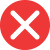30st

Incorrect spelling, explanation: this form, 30st, is a mistake because the suffix used to create ordinal numbers is the one that word forms of these numbers end with. Therefore thirtieth is 30th and thirty-second is 32nd. This means the only correct form here is 30th.30th

Correct spelling, explanation: as with most ordinal numbers, thirtieth (30th) in its number form is written with -th suffix after 30. It is due to the fact, that its word form thirtieth ends with -th; according to the rule, this is how we create suffixes for ordinal numbers. -st suffix is used only with number 1, as in first. Therefore 30th is the only correct form here, and 30st is mistaken.

Definition of 30th:
numeral, the ordinal number between 29th and 31st
This is a the 30th book I’ve read this year, and I’m very proud of myself.
I was 30th in the queue, so I waited for 3 hours.30nd

Incorrect spelling30rd

Incorrect spelling